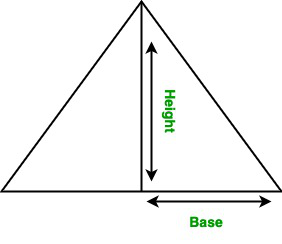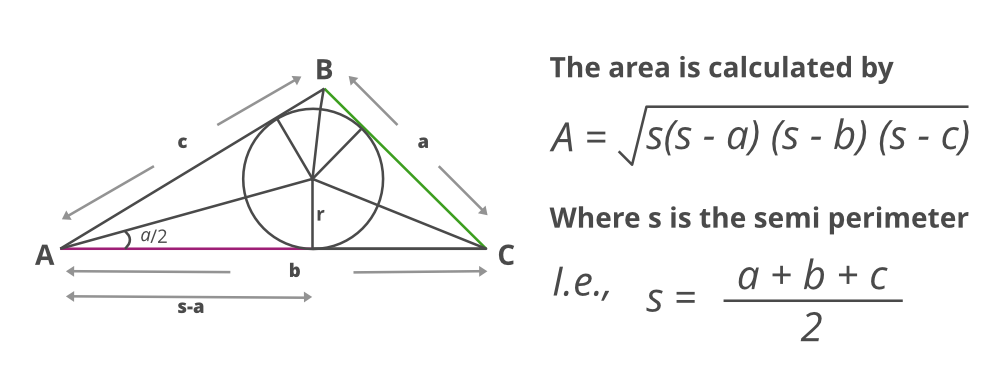Java Program to Find the Area of a Triangle

• Last Updated : 02 Dec, 2020

A triangle is a polygon. It has three edges and three vertices and each vertex from an angle. It is a closed 2-dimensional shape. In this article, we will learn how to find the area of the triangle.

There can be two possibilities while calculating the area of the triangle as per cases

• Using the height and base of the triangle
• Using the 3 sides of the triangle

Case 1: When the height and base of the triangle are given, then the area of the triangle is half the product of its base and height.Formula:

Area of triangle: Area = (height×base)/2

Example 1: Evaluation of area using base and height

Java

 // Java program to find the// area of the triangle  // Importing java librariesimport java.io.*;  class GFG {      // Function to calculate the    // area of the triangle    static double area(double h, double b)    {        // Function returning the value that is        // area of a triangle        return (h * b) / 2;    }      // Main driver code    public static void main(String[] args)    {        // Custom inputs- height and base values          // Height of the triangle        double h = 10;          // Base of the triangle        double b = 5;          // Calling area function and        // printing value corresponding area        System.out.println("Area of the triangle: "                           + area(h, b));    }}

Output:

Area of the triangle: 25.0

Case 2: When the three sides of the triangle are given

Now suppose if only sides are known to us then the above formula can not be applied. The area will be calculated using the dimensions of a triangle. This formula is popularly known as Heron’s formula.Algorithm:

1. The Semiperimeter of the triangle is calculated.
2. Product of semi meter with 3 values where these rest of values are the difference of sides from above semi perimeter calculated.
3. Square rooting the above value obtained from the computations gives the area of a triangle.

Example 2:

Java

 // Java program to find the area of// the triangle using Heron’s formula  // Importing java librariesimport java.io.*;  class GFG {      // Function to calculate the area where parameters    // passed are three sides of a triangle    static float area(float r, float s, float t)    {        // Condition check over sides of triangle        if (r < 0 || s < 0 || t < 0 || (r + s <= t)            || r + t <= s || s + t <= r)          // Length of sides must be positive and sum of        // any two sides must be smaller than third side          {            // print message if condition fails            System.out.println("Not a valid input");            System.exit(0);        }          /*else*/          // Finding Semi perimeter of the triangle        // using formula        float S = (r + s + t) / 2;          // Finding the area of the triangle        float A = (float)Math.sqrt(S * (S - r) * (S - s)                                   * (S - t));          // return area value        return A;    }      // Main driver code    public static void main(String[] args)    {        // custom inputs of sides of values          // Sides of the triangle        float r = 5.0f;        float s = 6.0f;        float t = 7.0f;          // Calling area function and        // printing the area of triangle        System.out.println("Area of the triangle: "                           + area(r, s, t));    }}

Output:

Area of the triangle: 14.6969385

My Personal Notes arrow_drop_up